Geometry

# Similar Polygons: Level 3 Challenges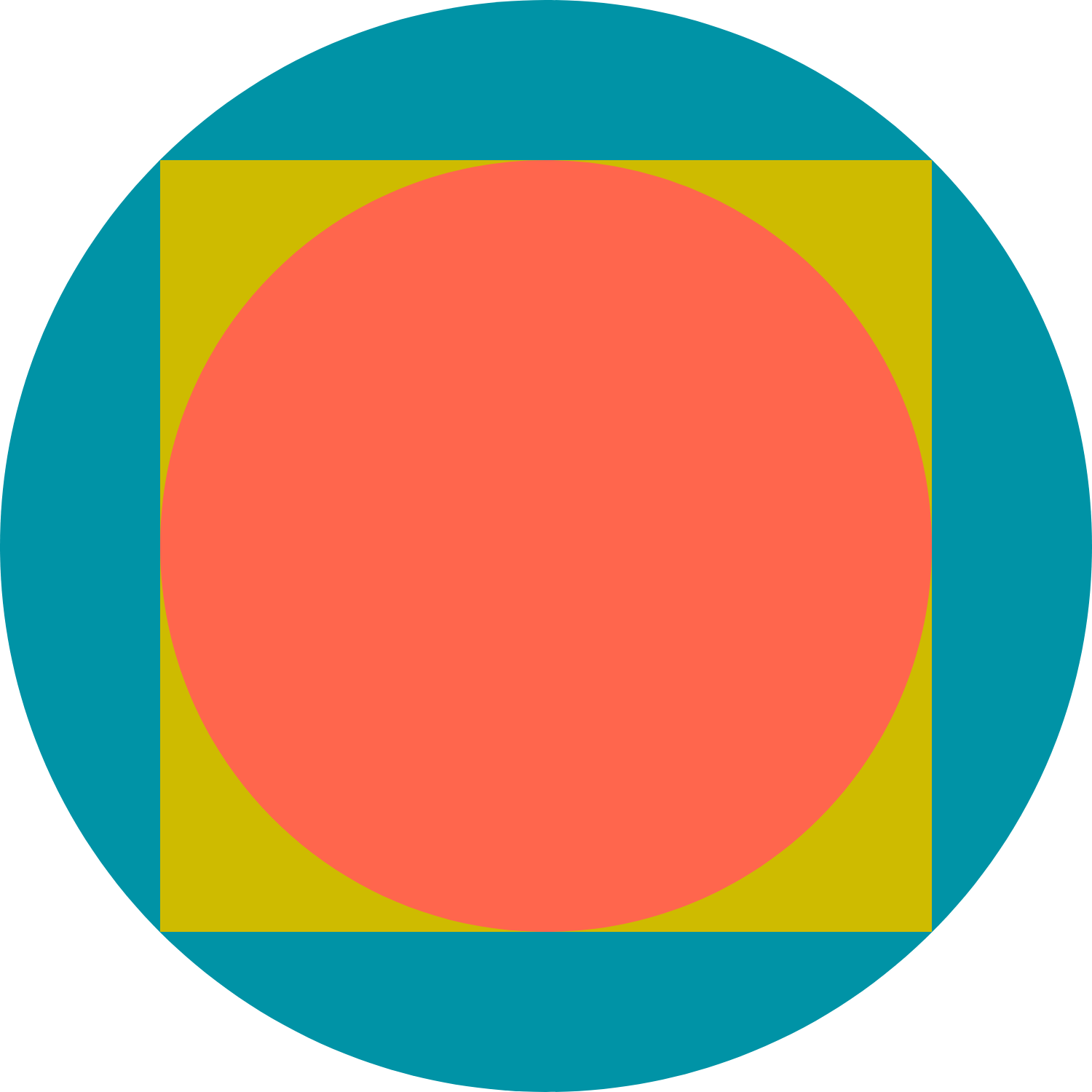A square is inscribed in a circle and a circle is inscribed in that square. What will be the ratio of the area of the bigger circle to that of the smaller circle?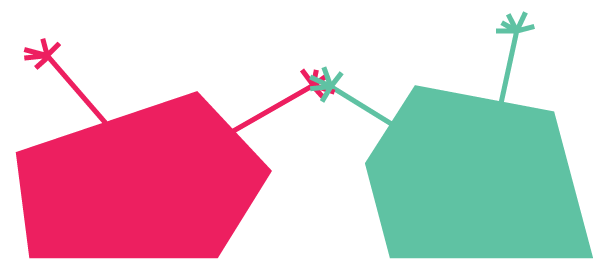The sum of the areas of two similar polygons is 65 square units. If their perimeters are 12 units and 18 units, respectively, what is the area of the larger polygon?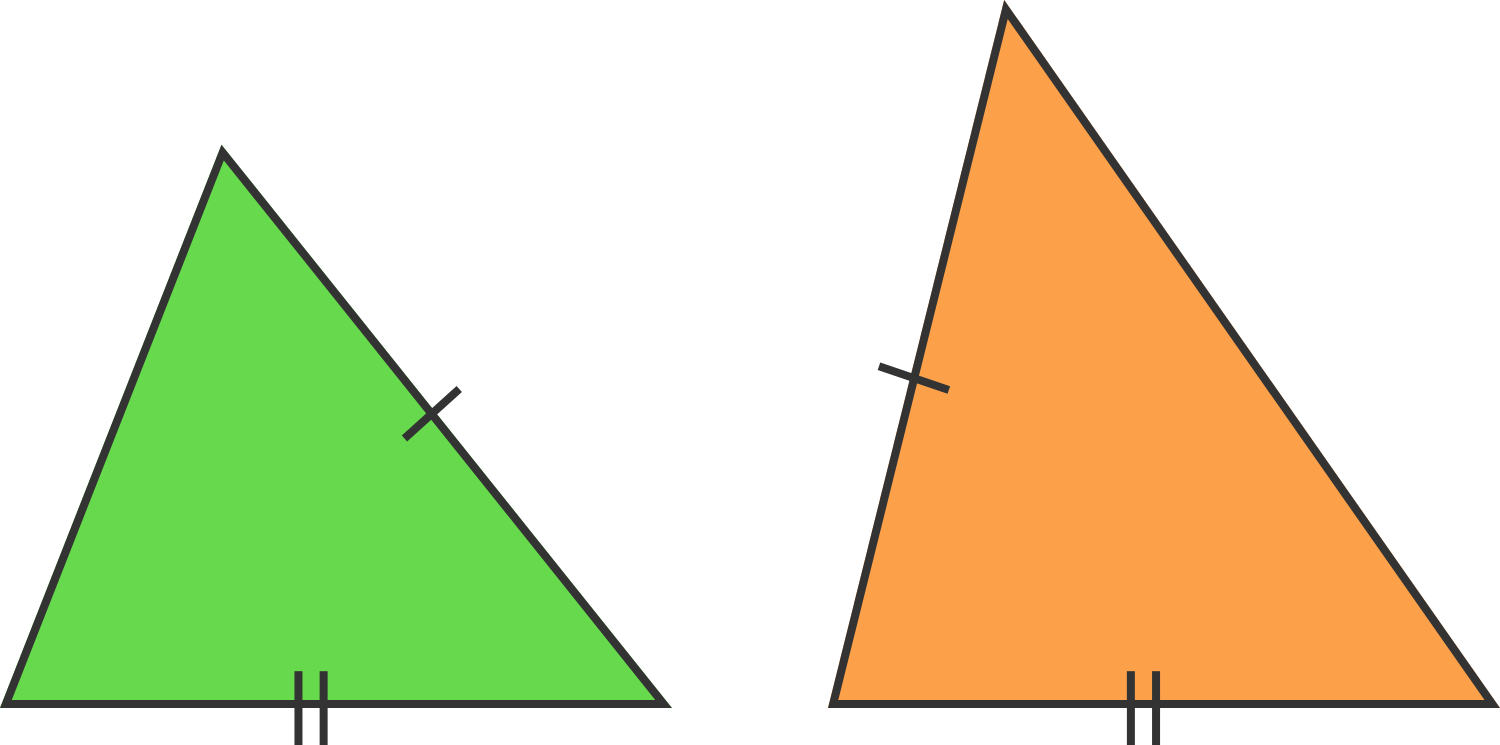Two triangles have integral side lengths, with all sides being less than 50. They are similar but not congruent, and the smaller triangle has two side lengths identical with the larger triangle.

What is the sum of the side lengths of the larger triangle?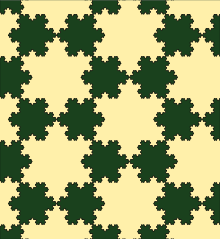If a tan snowflake has area $T$, and a dark green snowflake has area $G$, what is $\frac{T}{G}$?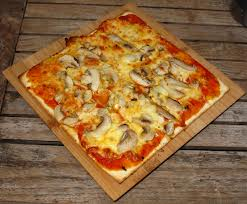Usually, pizzas are circular and they come in boxes with a square base. The circular pizza covers some percentage of area of the square base of the box. Let's say someone makes a square pizza and puts it in a box with a circular base. Will the square pizza cover more percentage of area in the box with circular base than the circular pizza in the box with square base?

Assume that both the pizzas fit perfectly inside their respective boxes.

×